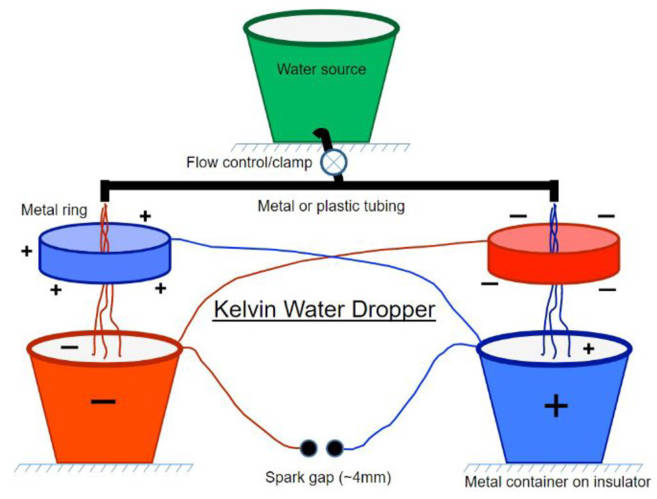## MIT电和磁

(2) 赵凯华-电磁学讲义
(3) TED-电学词汇历史
(4) 电和磁—hyperphysics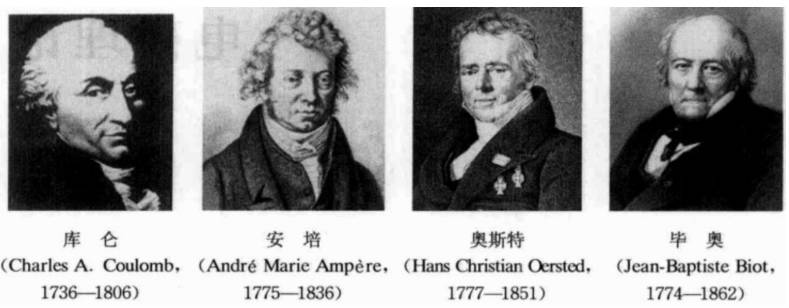### 是什么将世界连在一起

In ancient times, amber was noticed to attract light bits of matter. 比如摩擦琥珀(amber)可以吸引树叶。希腊语的琥珀就是elektron。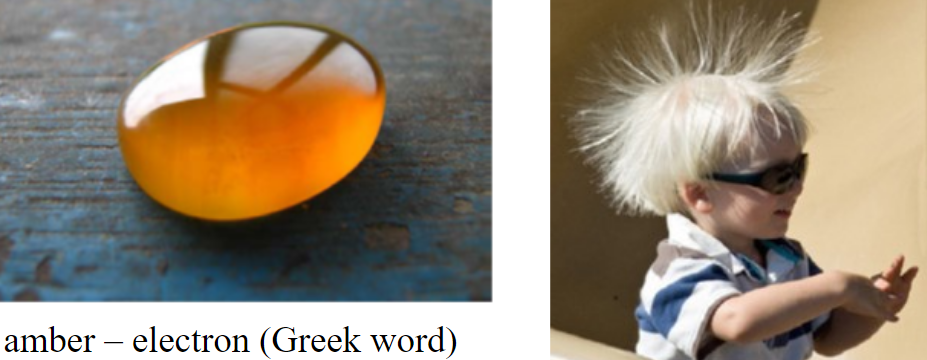(1) 出门前去洗个手，或者先把手放墙上抹一下去除静电，还有尽量不穿化纤的衣服。
(2) 小金属器件（如钥匙）、棉抹布等先触碰大门、门把、水龙头、椅背、床栏等消除静电，此过程即使有放电，手也不会感到疼痛，因为放电的接触点不是手上皮肤的某个点。
(3) 穿全棉的内衣。
(4) 冬天在暖气下放置一盆水，用一条旧毛巾(或吸水好的布)，一头放在水里，一头搭在暖气上，这样一昼夜可以向屋里蒸发大约三升水。

(1) 如果气球导电，很显然，存在电荷移动，靠近带电棒的一侧带负电，远离的一侧带正电，气球被吸引；
(2) 如果气球不导电，虽然电子不能自由移动，但是仍存在极化作用，也就是说靠近带电棒的一侧的气球的原子层，它们的电子都围绕各自的原子核运动，但是现在电子在靠近带电棒的一侧出现的概率更大，换句话说电子云发生了形变，趋向于靠近带正电的棒子，故同样出现气球靠近带电棒的情况。
(3) 导电的气球，如果接地，很容易把自身带的电放走；不导电的气球接地，很难完全放电完全。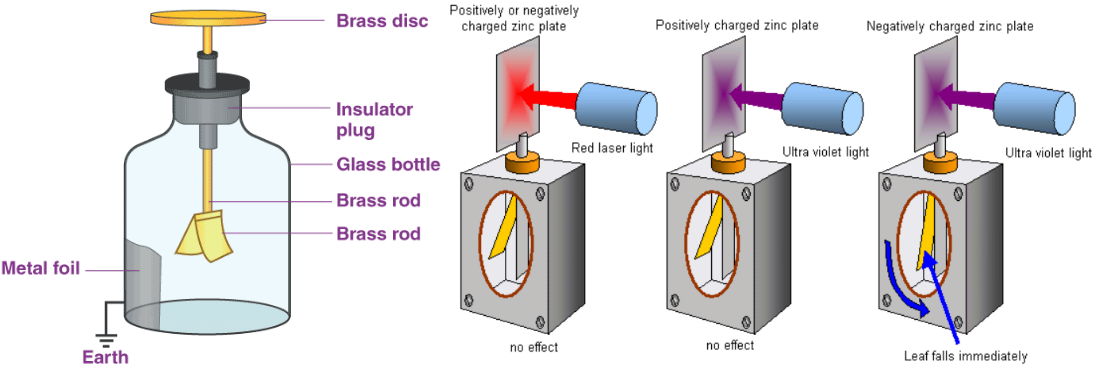• no electrons were emitted from the plate if it was positive
• the number of electrons emitted per second depended on the intensity of the incident radiation
• the energy of the electrons depended on the frequency of the incident radiation
• there was a minimum frequency below which no electrons were emitted no matter how long radiation fell on the surface

### 电场和偶极子

(1) 库仑力比引力大多了，大$$10^{35}$$个数量级；
(2) 电荷有正负之分，同性相斥，异性相吸，两个相反的电荷相遇会彼此中和。

• 单个电荷$$q$$是电单极子(electric monopole)，它的电场随$$r^{-2}$$减小
• 两个电量相等、符号相反的电荷形成一个偶极子(dipole)，不存在电场为零的点，远距离处电场随$$r^{-3}$$减小
• 两个相等、相反的偶极子组成一个四极子(quadrupole)，在远距离处电场随$$r^{-4}$$减小
• 两个近邻的电荷，一个带$$+3$$的电，一个带$$-1$$的电，那么很远离这两个电荷的地方，受到的电场力可以将两个电荷等效为带$$+2$$的电荷，电场强度随距离的衰减仍然满足平方反比定律；另外也存在电场为$$0$$的特殊点。
• 还可以继续构建更多的多极子。如果你把这些不同的极子混合起来放进一个“黑盒子”里，在盒子外面可以产生任何类型的电场，如果不打开盒子就不可能知道盒子里面到底装的是什么。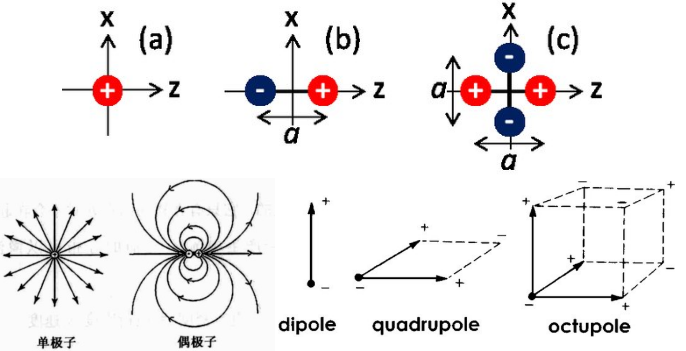• 举例：比如一个原子，没有电场作用下，(电介质，或者说绝缘体)正负电荷的中心是重叠的，但是在电场中，电子会更倾向于处在电场线的上游，原子核更倾向于处在电场线下游的方向，因此就会出现正负电荷中心不重合的情况，那么就形成了偶极子。另外，当一段金属线(无线电发射天线)里电子做周期性运动，使得金属线的两端交替地带正、负电荷，形成振荡偶极子
• 电偶极子的场强与距离的三次方$$r^3$$成反比，比点电荷随距离的衰减速度快得多。
• 通过简单计算(计算细节见《电磁学—赵凯华》，电偶极子的场强公式
两个电荷延长线上$$E \approx \displaystyle\frac{1}{4 \pi \varepsilon_{0}} \frac{2 p}{r^{3}}$$，中垂面上$$E \approx \displaystyle\frac{1}{4 \pi \varepsilon_{0}} \frac{p}{r^{3}}$$。
• 电偶极子在均匀电场中所受的力矩：$$\boldsymbol{L}=q \boldsymbol{l} \times \boldsymbol{E}$$，电偶极矩$$p=q \boldsymbol{l}$$，从负电荷指向正电荷。
• 演示实验：
• 两个分别带$$+$$电和$$-$$电的金属球用绝缘棒连接，就可以形成一个偶极子的演示实验，将这个偶极子放在一个电场中(范式起电机)，绝缘棒中间位置用个绳子吊着，可以发现由于带正电的小球和带负电的小球的受力方向相反，所以偶极子会来回摆动，然后由于阻尼(damping)作用，摆动幅度越来越小。
•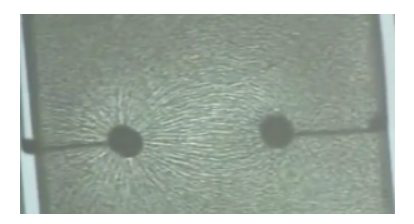右图是演示偶极子在电场中的状态。左右两边分别是带正负电荷的电极，然后整个是在油里面，油里面分布有很多草种子，这些种子在电场作用下，会类似磁铁砂在磁场中的分布一样，呈现一定方向的排列，展示出电场的形状，锥形草种子稳定的时候，电场线下游的一端带正电，另一端带负电，锥形草的方向就是电场线的方向。

### 电通量和高斯定律

$$\Phi_{E}={\large\bigcirc}\kern-1.55em\iint \mathbf{E} \cdot d \mathbf{S}=\frac { Q }{ \varepsilon _{ 0 } }$$微分形式$$\nabla \cdot \mathbf{E}=\frac{\rho}{\epsilon_{0}}$$

1. $$\rho$$是体电荷密度。对于积分形式等式右边我们取特殊情况，也就是正电荷$$Q$$处在球中心来计算，球面每点的场强都相等，都和对应的微元面积垂直，所以电场强度乘以球表面的面积即可得到等式右边。
2.散度$$\operatorname{div} \mathbf{F}=\nabla \cdot \mathbf{F}=\left(\displaystyle\frac{\partial}{\partial x}, \displaystyle\frac{\partial}{\partial y}, \displaystyle\frac{\partial}{\partial z}\right) \cdot\left(F_{x}, F_{y}, F_{z}\right)=\displaystyle\frac{\partial F_{x}}{\partial x}+\displaystyle\frac{\partial F_{y}}{\partial y}+\displaystyle\frac{\partial F_{z}}{\partial z}$$
3. 库伦常数和真空介电常数之间的关系$$k_{e}=\displaystyle\frac{1}{4 \pi \varepsilon_{0}}$$
4.$$E=\displaystyle\frac{Q}{4 \pi r^{2} \varepsilon_{0}}$$，如果是介质中的点电荷，那么这里的真空介电常数将会由更大的介电常数(相对介电常数乘以真空介电常数$$\varepsilon_0$$)替代，相同距离的地方的电场强度显然更小。
5.$$E=\displaystyle\frac{Q}{4 \pi r^{2} \varepsilon_{0}}$$单位面积包裹(这里是直观的球体，对于平板电容器$$\displaystyle\frac{Q}{A}=\varepsilon_{0} E$$)的电荷数和产生的电场之间的比例常数(必须是任意位置电场线和面积微元垂直)是$$\varepsilon_0$$。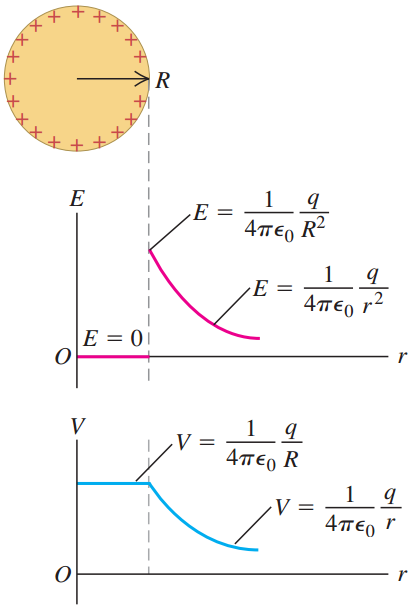(外表面)均匀带电的空金属球(如右图)内部，电场强度都为$$0$$，因为取球内任意一点作等圆心的球面，球面包含的电荷为$$0$$，故每处的电场强度都是$$0$$。同理，球外任意一点的场强也可以通过作高斯面的方法求得，影响场强大小的因素是里面包含的电荷量，而与电荷到底是怎样的空间分布无关。注意，事实上带电金属球的电荷只能分布在表面，可以用反证法说明。

• 球形对称；
• 柱对称；
• 无线平面分布。

hollow行星，或者说在一个质量均匀分布的球体里面，按照相同的球心位置挖出一个球，那么中空的部分重力场为$$0$$。牛顿还研究了这样一个问题，假设地球是质量均匀分布的完美球体，那么地球外任意一点(即使离地球不远)的引力场(地球造成的)很容易计算，因为引力也是平方反比，也可以用高斯定律处理，也就是说，可以把地球看成一个质点，所有的质量都集中在地球的球心。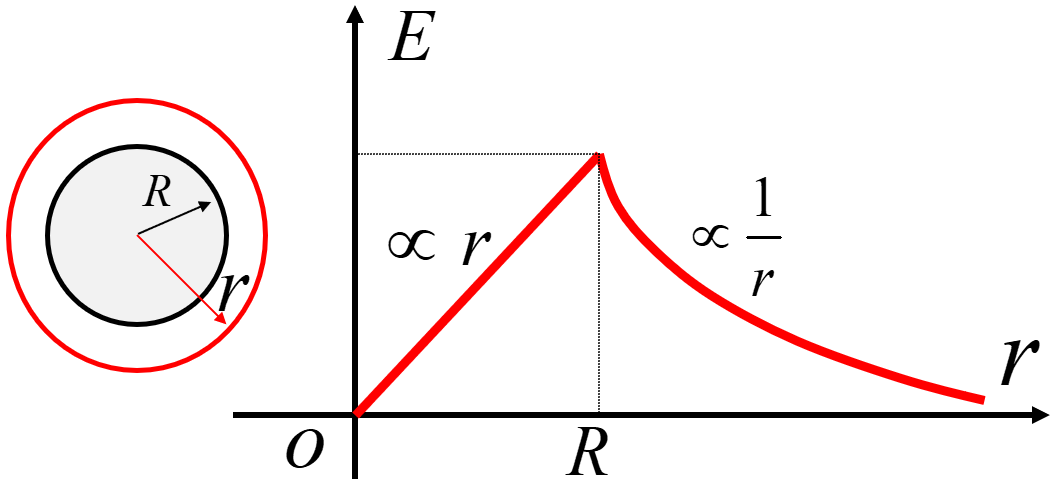柱对称电场强度：即均匀带电圆柱体的电场分布。注意这里的电荷是均匀分布在圆柱体中，表面有，内部也有。如果这个圆柱体是导体的话，净电荷只分布在表面，所以显然这里的情况不是导体。求解电场分为两种情况，圆柱外的电场，以及圆柱内的电场。取高斯面的时候，相当于围绕轴取一个圆柱体，由于对称性使得圆柱体的上下表面的电通量为零，所以只需要考虑该圆柱体的测面通量，利用高斯定理即可求解。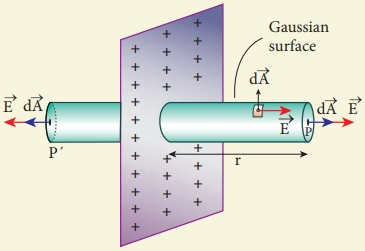带电无线平面(charged infinite planar sheet)，假设单位面积的带电量为$$σ=Q/A$$，那么任意一点的场强为$$E=\frac { \sigma }{ 2{ \varepsilon }_{ 0 } }$$与该点到带电平面的距离无关，关键在于高斯面的选取。图中只有两侧圆形有电通量。计算方法如下：$$2 E \int_P d A=\frac{\sigma A}{\varepsilon_0}$$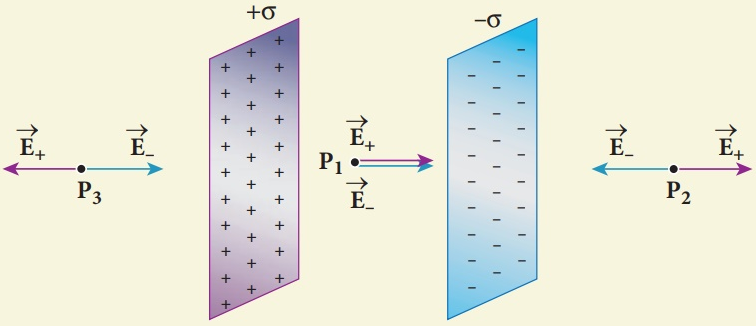### 静电势能和电势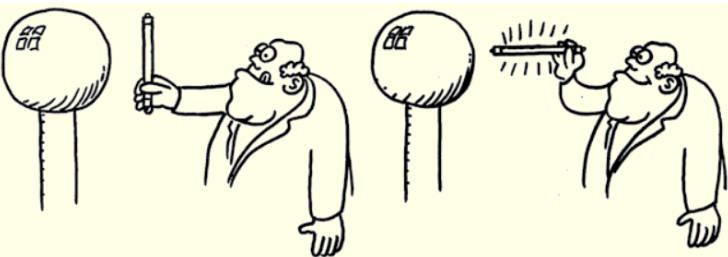### 静电屏蔽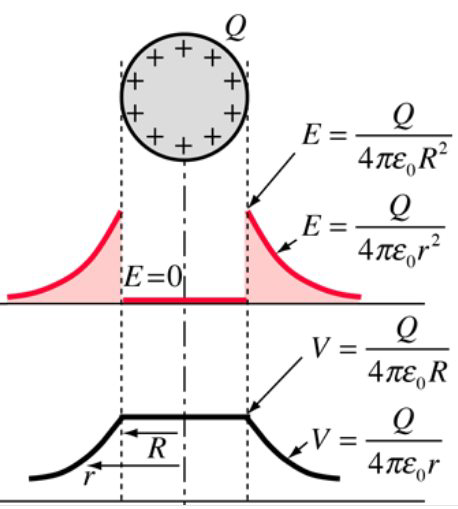带电中空球电荷分布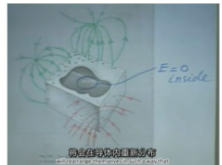大自然的神奇之处：将一个不带电的封闭的铁盒子放在一个电场中，盒子马上由于电场的左右产生极化，一侧带正电，另一侧带负电，而且电荷的分布会使得盒子内部的电场强度处处为零，盒子外部所有的电场线都是垂直于盒子表面(保证盒子是个等势体)。这种电荷的重新分布是在一瞬间完成的，而且遵循所有的物理定律。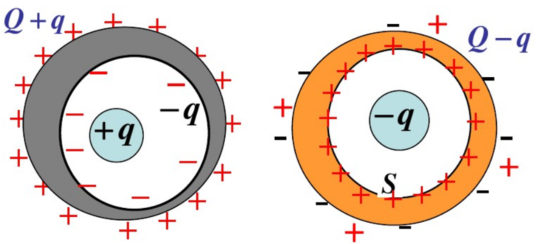腔体内部有电荷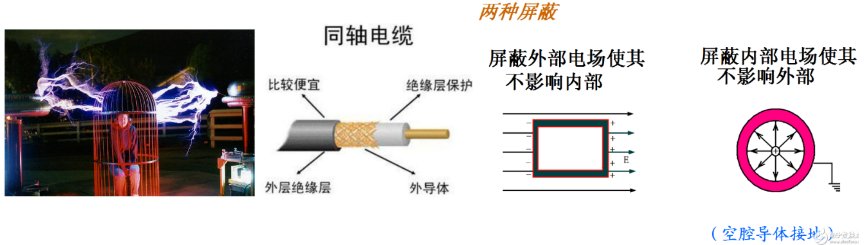(1)  同轴电缆就是利用了法拉第笼的静电屏蔽效应;
(2)  汽车、飞机等交通工具中的人是不会被雷击的;
(3)  如果电梯内没有中继器的话，那么当电梯关上的时候，里面任何电子讯号也收不到。
(4)  为防止干扰，一些精密仪器需放在笼内才可进行运作或量测。

### 高压击穿和闪电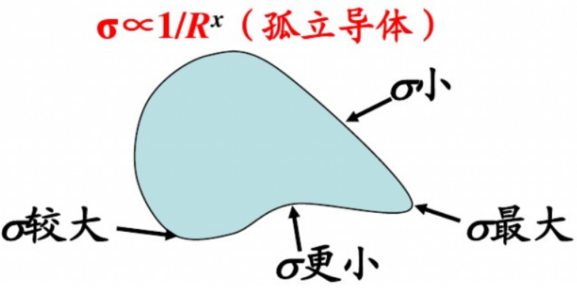Dependence of Charge Density on Surface Curvature of an Isolated Conductor 对于孤立导体：表面上的电荷密度的大小与该处表面的曲率有关。

• 曲率较大，表面尖而凸出部分，电荷面密度较大；
• 曲率较小，表面比较平坦部分，电荷面密度较小；
• 曲率为负，表面凹进去的部分，电荷面密度最小。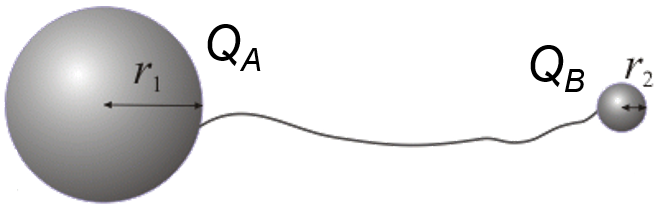Electrically connected charged balls

Townsend avalanche(雪崩avalanche [ˈævəlɑːnʃ] )就是一次电击穿(electric breakdown)，你就可以看到电火花(spark)。The Townsend discharge or Townsend avalanche is a gas ionisation process where free electrons are accelerated by an electric field, collide (/ kəˈlaɪd /) with gas molecules, and consequently free additional electrons. Those electrons are in turn accelerated and free additional electrons. The result is an avalanche multiplication that permits electrical conduction through the gas. The discharge requires a source of free electrons and a significant electric field; without both, the phenomenon does not occur.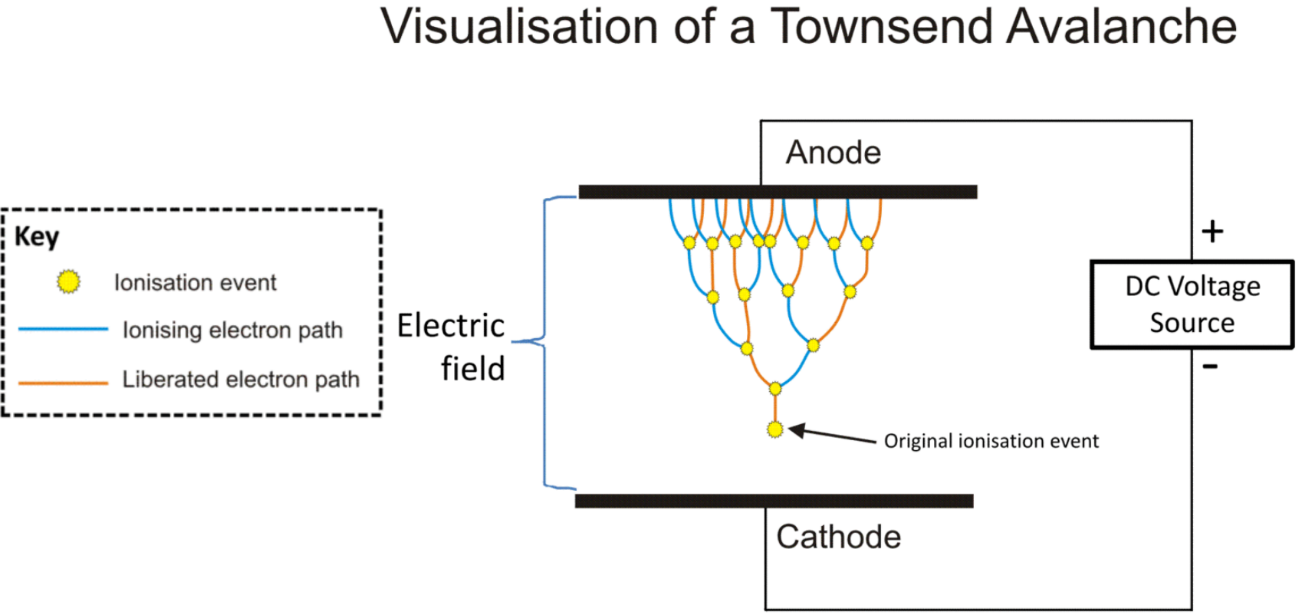### 电容和场能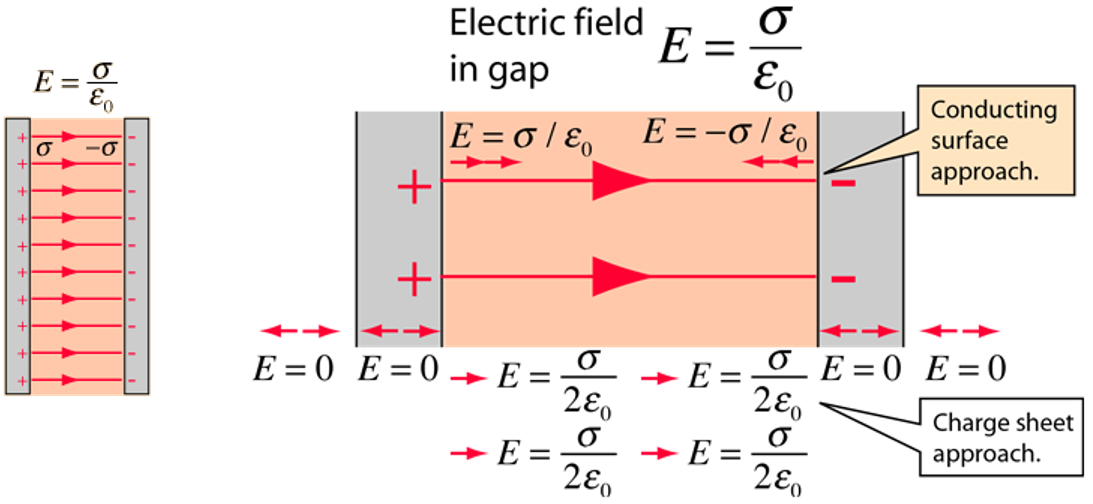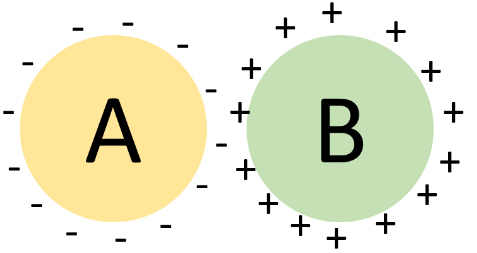电势的定义是，单位电荷到指定位置所做的功，于是对于独立的B来说$$C_B=\displaystyle\frac{Q_B}{V_B}$$。如果将A放在B旁边，由于A的存在，单位电荷达到B所做的功会减小，所以B的电势$$V_B$$会变小，则B的电容$$C_B$$就变大了。注意此时我们把$$C_B$$叫作B的电容是不准确的，我们把它看作是A存在的情况下B的电容，而不是单独考虑B。

• 与面积成正比比较好理解，面积越大所能携带电荷越多；
• 与距离成反比，距离越近，负电荷板把正电荷板的电势降低越多(因靠的越近，单位电荷从无限远处移动到正电荷板所需的功越小)，根据电容是电荷量与电势的比值，电势越小，电容就会越大。或者这样想，因为带电量固定后，电容中的电场强度就固定了，因此距离越小，两个板之间电势差就越小，不变的电量除以变小的电势差，于是电容就变大了。

• 闪光灯
• 飞机/机场灯塔的警示信号
• 相机闪光灯，高速摄影闪光灯
• 测量电机转速
• 电蚊拍
• AED(除颤器)
• 频闪观测仪

• MLCC (Multi layer Ceramic Capacitors)，多层陶瓷电容，也叫独石电容，用于小功率的滤波，旁路，耦合；
• 瓷片电容
• 铝电解电容：贴片和插件两种形式，内部电介质为铝电解液，区分正负极，用于低频直流电场合，最主要的作用是给电源滤波；
• 钽电容：耐压不行，价格高，钽电容对高低频信号的响应都较好，频率适用范围宽，等效串联电阻小；
• 薄膜电容

### 电介质及极化

approach it in a very cold-blooded way 用非常冷酷的方式推导
mica [ˈmaɪkə] 云母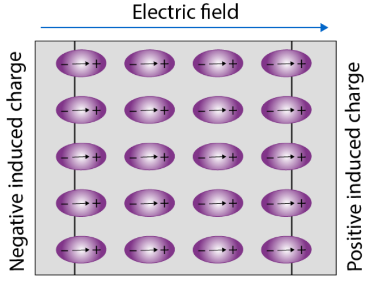电介质的极化，对于绝缘体来说，在外界电场作用下，虽然没有自由电子的移动，但是原子的电子云会被一定程度上拉长变形，于是正负电荷中心不重叠，形成了偶极子，在绝缘体的上下表面就会分别聚集电性相反的电荷，形成内部的一个感应电场。在电容的中间插入电介质，电容上的电荷称作自由电荷，电介质上的电荷称作感应电荷(induced charges，对应的有感应电场)。

\begin{aligned}
&E_{\text {free}}=\frac{\sigma_{\text {free}}}{\varepsilon_{0}}, E_{\text {indu}}=\frac{\sigma_{\text {indu}}}{\varepsilon_{0}}\\
&\vec{E}_{\text {net}}=\vec{E}_{\text {free}}+\vec{E}_{\text {indu}}=E_{\text {free}}-E_{\text {indu}}\\
&\sigma_{\text {indu}}=b \sigma_{\text {free}},(b<1)\Rightarrow E_{\text {indu}}=b E_{\text {free}}\\
&E_{\text {net}}=(1-b) E_{\text {free}}\end{aligned}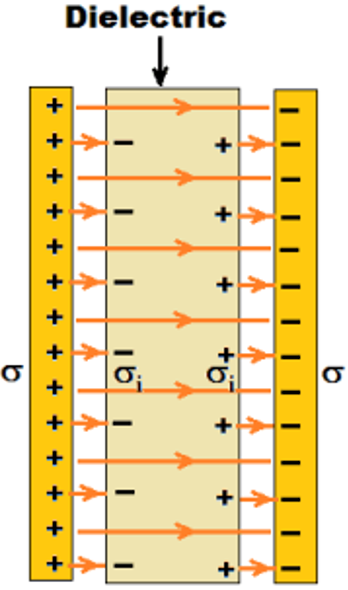$$(1-b)$$我们叫做$${ 1}/{ \varepsilon_r}$$，$$\varepsilon_r$$称作【相对介电常数】(relative permittivity，或者dielectric constant)。没有量纲的数值。解释一下就是：电容充电后，和电源断开，然后插入电介质，由于电介质产生感应电场，电容内部的电场强度会被削弱，不插入电介质时候的电场强度和插入电介质后的电场强度(net)之比就是相对介电常数，另外很容易知道插入电介质，电容两端的电势差会降低。$$\varepsilon=\varepsilon_0 \varepsilon_r$$为电容率(absolute permittivity，或者直接简写为permittivity)。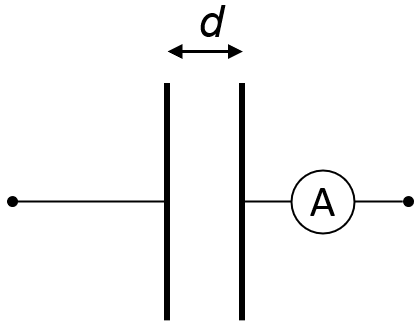平行板电容器充电后断电，再插入电介质：
(1) 电流表不动，表明两极板各自所带电荷$$Q$$没变—极板没有放电也没有充电；
(2) $$V=Ed$$，$$d$$不变，$$E$$变小了，所以两极板间电压变小；
(3) $$Q = VC$$，$$Q$$没变，$$V$$变小，所以两极板的电容变大了。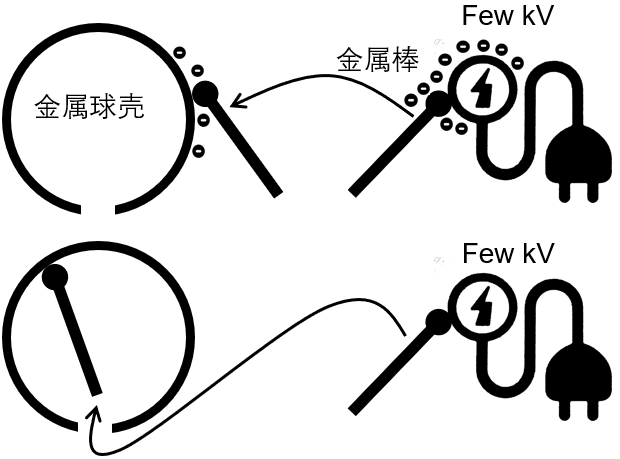(1) 如果每次用金属棒接触电源(成为一个等势体)，然后再将金属棒贴在金属球壳的外表面，由于金属棒的电势比金属球壳高，所以电子会从金属棒流向金属球壳，然后再将金属棒接触电源，不断重复这个过程，于是金属球壳上的电子越来越多，直到金属球壳的电势和电源的电势相等，我们就无法再往球壳上转移电子了。
(2) MIT教授Van de Graaff发现，在(1)的终态的基础上，可以将接触了电源的金属棒移动到金属球壳的内部，这个过程其实是对电子做功，使得金属棒的电势比金属球壳的内表面高，如果金属棒接触金属球壳内表面，那么电子就会从金属棒转移到金属球壳内表面，然后快速转移到外表面。不断重复这个过程，就可以使金属球壳产生$$30$$万伏的电压。这就是范氏起电机】(Van de Graaff generator)的原理。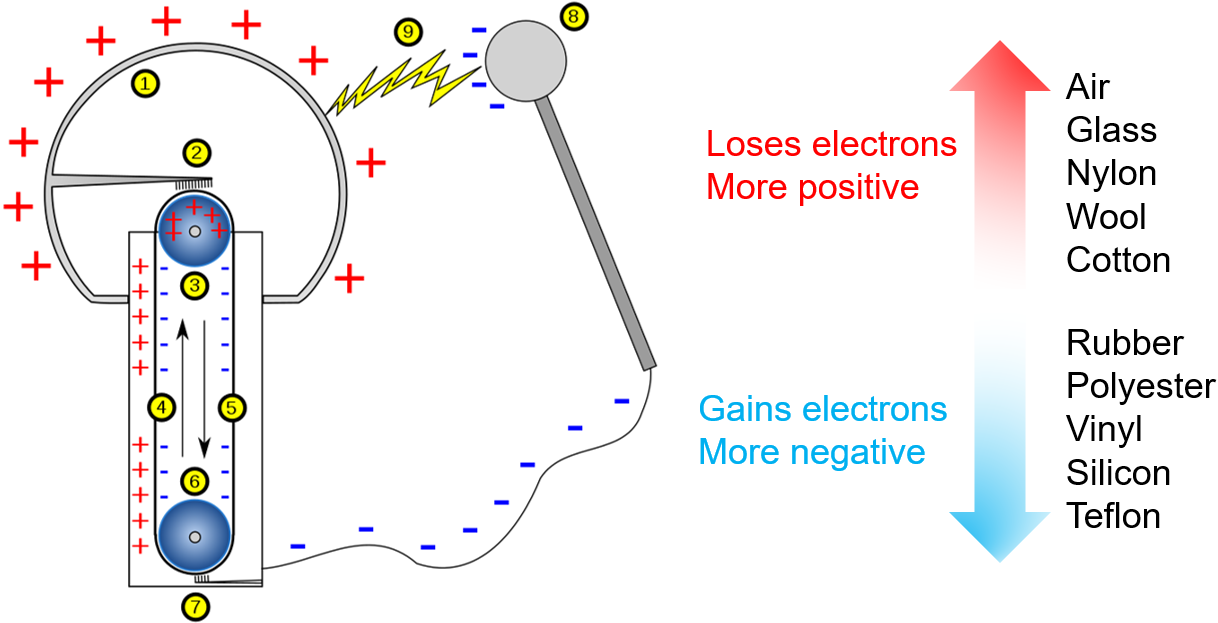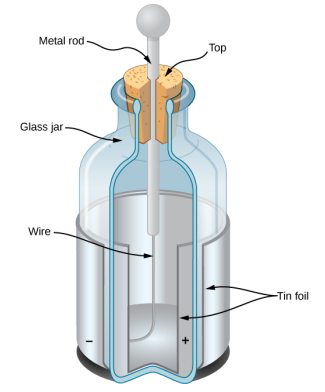莱顿瓶】(Leyden jar )，是一种用以储存静电的装置。作为原始形式的电容器，莱顿瓶曾被用来作为电学实验的供电来源，也是电学研究的重大基础。莱顿瓶的发明，标志着对电的本质和特性进行研究的开始。至今，莱顿瓶任然在演示静电学原理时被使用。利用了莱顿瓶把天上的闪电引入到莱顿瓶中，证实了闪电和日常生活中的摩擦起电实质上是同一类东西，相当于把二者统一起来，牛顿也是把天体之间的引力和苹果落到地上的重力统一为万有引力。

### 电流、电阻率和欧姆定律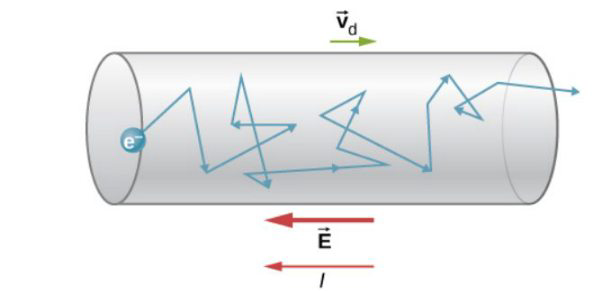drift velocity漂移速度 $$v_d=a \tau=\displaystyle\frac{e E}{m_e} \tau$$。电场加强时，漂移速度会增加；间隔增大，加速时间变长，漂移速度也会增大。可以由此推出欧姆定律(其中$$R =l/ \sigma A$$，$$n$$为体电荷密度)$$I=v_dAne =\displaystyle\frac{e^2n\tau}{m_e} AE= \sigma AE=\frac {\sigma AV }{l}= \frac {V}{R}$$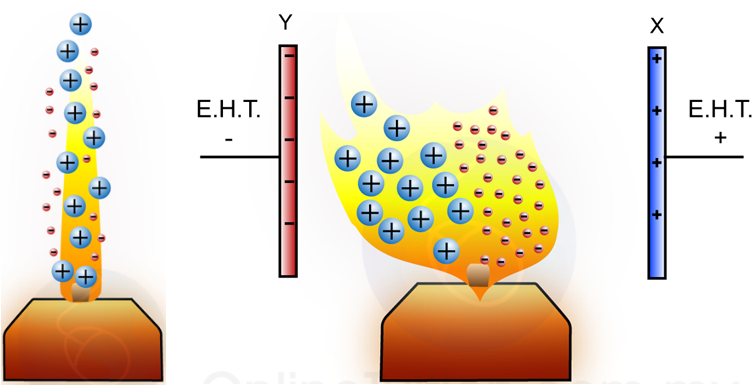The heat of the candle flame removes electrons from the air molecules around it, and therefore ionised the molecule. 如果在蜡烛两侧的金属板上连接Extra High Tension (E.H.T.) power supply，那么会出现如图所示的形状。The spreading of the flame is not symmetry. This is because the positive ions are much bigger than the negative ions; it will collide with the other air molecule and bring more air molecule towards the negative plate. 火焰至少部分有处在等离子体状态。

### 电池和电动势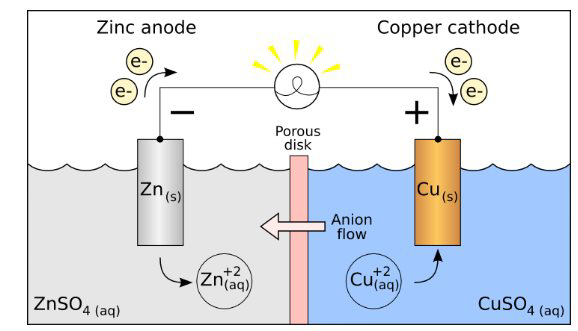The electrochemical reaction is$$\mathrm{Zn}(\mathrm{s})+\mathrm{Cu}^{2+}(\mathrm{aq}) \longrightarrow \mathrm{Zn}^{2+}(\mathrm{aq})+\mathrm{Cu}(\mathrm{s})$$当右边铜溶液浓度变淡(镀铜)，或者说锌溶液变浓(锌溶解)到一定程度，电池就停止工作。我们可以利用外接电源提供一个反方向的电流，使整个化学反应逆转。汽车蓄电池的原理和这个实验完全一样，只是用铅和氧化铅替代锌和铜，也是用到硫酸。常见的镍镉电池也一样，他们可以充电。

short out a battery可以得到最大电流$$I_{\max }=\displaystyle\frac{E M F}{r_i}$$，其中$$r_i$$为电源内阻。